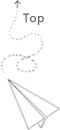# OpenGL 筆記 - Camera

OpenGL 中模擬相機的方法是，把場景(整個世界)照相機想移動的相反方向移動，來模擬出相機的感覺。

## View(Camera) SpaceView Space 是指把相機當作原點的座標，一個相機可以用以下三個東西定義出來:

• 相機(Camera)
• 位置
• 觀察的方向
• 相機頭頂的方向向量

• 位置
• 觀察的方向
• 右向量(對於相機)
• 這裡會用世界的 $y$ 軸向量(向上)跟 觀察方向做外積
• 拿到的向量會朝向 View Space 的 $+x$ ，也就是相機的右邊
• 上向量(對於相機)
• 我們已經有 View Space 的 $x$ 軸和 $z$ 軸的向量

### Look At 矩陣

$$LookAt = \begin{bmatrix} \color{red}{R_x} & \color{red}{R_y} & \color{red}{R_z} & 0 \\ \color{green}{U_x} & \color{green}{U_y} & \color{green}{U_z} & 0 \\ \color{blue}{D_x} & \color{blue}{D_y} & \color{blue}{D_z} & 0 \\ 0 & 0 & 0 & 1 \end{bmatrix} * \begin{bmatrix} 1 & 0 & 0 & -\color{purple}{P_x} \\ 0 & 1 & 0 & -\color{purple}{P_y} \\ 0 & 0 & 1 & -\color{purple}{P_z} \\ 0 & 0 & 0 & 1 \end{bmatrix}$$

$\color{Red}{R}$ 是右向量、$\color{Green}{U}$是上向量、$\color{Blue}{D}$是方向向量，$\color{purple}{P}$ 是相機位置，注意位移是相反方向，是因為前面提到我們希望將世界往相機的相反方向移動(因為 OpenGL 的相機在 $(0, 0, 0)$)

• GLM 提供了直接產生 Look At Matrix 的實用功能，只要提供相機位置、觀察目標、跟世界空間的上向量## 自由移動

lookAt 變成了:## 視角移動

### 歐拉角### 滑鼠輸入

• 計算滑鼠位置的偏移量(相對上個 frame)
• 把偏移量加到 pitchyaw
• 限制最大跟最小值
• 計算方向向量• 隱藏滑鼠游標

## 視角縮放## Lab

### 試著自己實作看看 lookAt()

• OpenGL 矩陣是 Column-major，所以 LookAt 公式要改寫成

$$LookAt = \begin{bmatrix} \color{red}{R_x} & \color{green}{U_x} & \color{blue}{D_x} & 0 \\ \color{red}{R_y} & \color{green}{U_y} & \color{blue}{D_y} & 0 \\ \color{red}{R_z} & \color{green}{U_z} & \color{blue}{D_z} & 0 \\ 0 & 0 & 0 & 1 \end{bmatrix} * \begin{bmatrix} 1 & 0 & 0 & 0 \\ 0 & 1 & 0 & 0 \\ 0 & 0 & 1 & 0 \\ -\color{purple}{P_x} & -\color{purple}{P_y} & -\color{purple}{P_z} & 1 \end{bmatrix}$$## 推薦文章